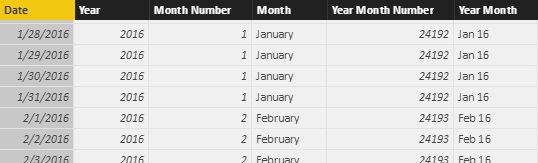Topic: Power Pivot

58 articles of 244 posts
article –

Values such as inventory and account balance, usually calculated from a snapshot table, require the use of semi-additive measures. This article describes how to implement these calculations in DAX according to your specific requirements.  Read more

article –

Understanding numeric data type conversions in DAX

This article describes how DAX automatically converts data types in arithmetic operations. These small details can cause and explain differences in results when using the same operations in other languages.  Read more

article –

Optimizing duplicated DAX expressions using variables

This article describes how to use variables to optimize the performance of DAX expressions containing multiple instances of the same measure or the same sub-expression.  Read more

article –

Context Transition and Filters in CALCULATE

This article explains how the context transition interacts with the filter arguments of a CALCULATE function in DAX. This is important in order to avoid unexpected results with complex calculations made in filter arguments.  Read more

article –

How to handle BLANK in DAX measures

This article describes a counterintuitive behavior of BLANK in DAX measures affecting Power BI, Analysis Services, and Power Pivot. That behavior could cause mistakes in a report using alternate expressions of the same calculation. Indeed, these expressions are not equivalent…  Read more

article –

Using KEEPFILTERS in DAX

This article explains how to use KEEPFILTERS to intersect instead of overriding an existing filter context in DAX, simplifying the code and improving performance.  Read more

article –

Storage differences between calculated columns and calculated tables

This article compares the differences between calculated columns and calculated tables from a processing and storage point of view.  Read more

article –

Measuring the dictionary size of a column correctly

This article explains how to correctly measure the dictionary size of a column in a model created in Power BI, Power Pivot, or Analysis Services Tabular.  Read morearticle –